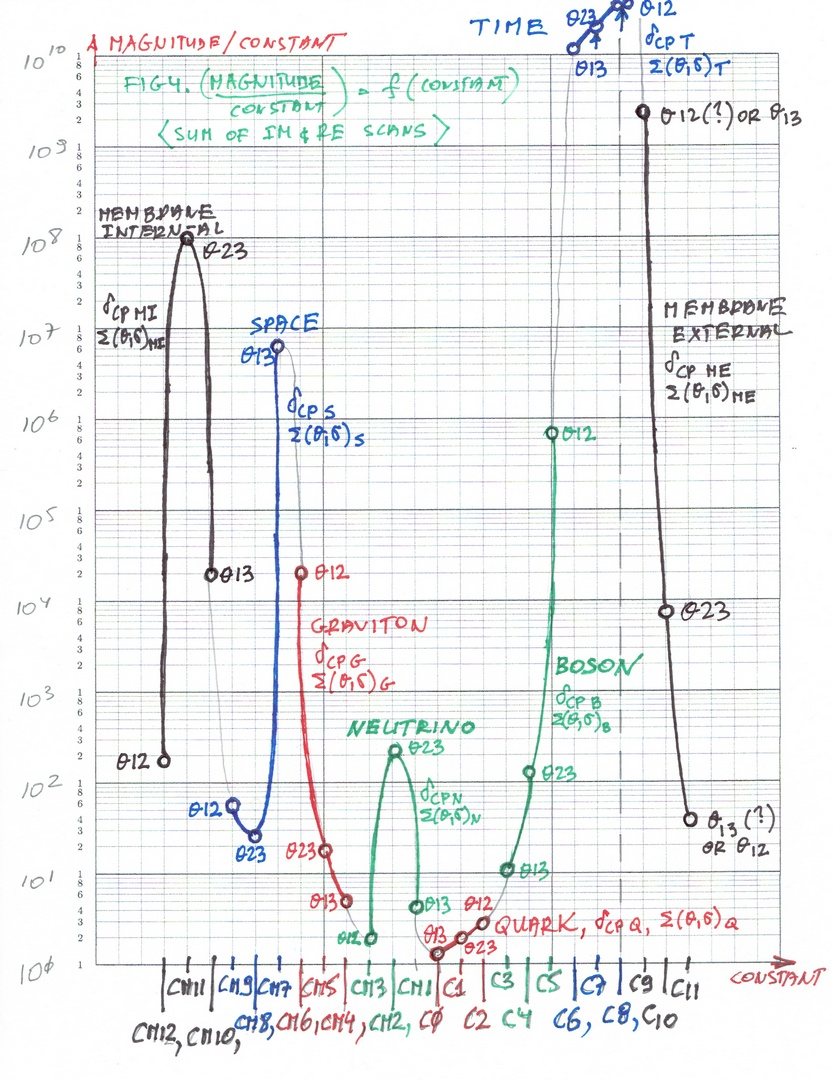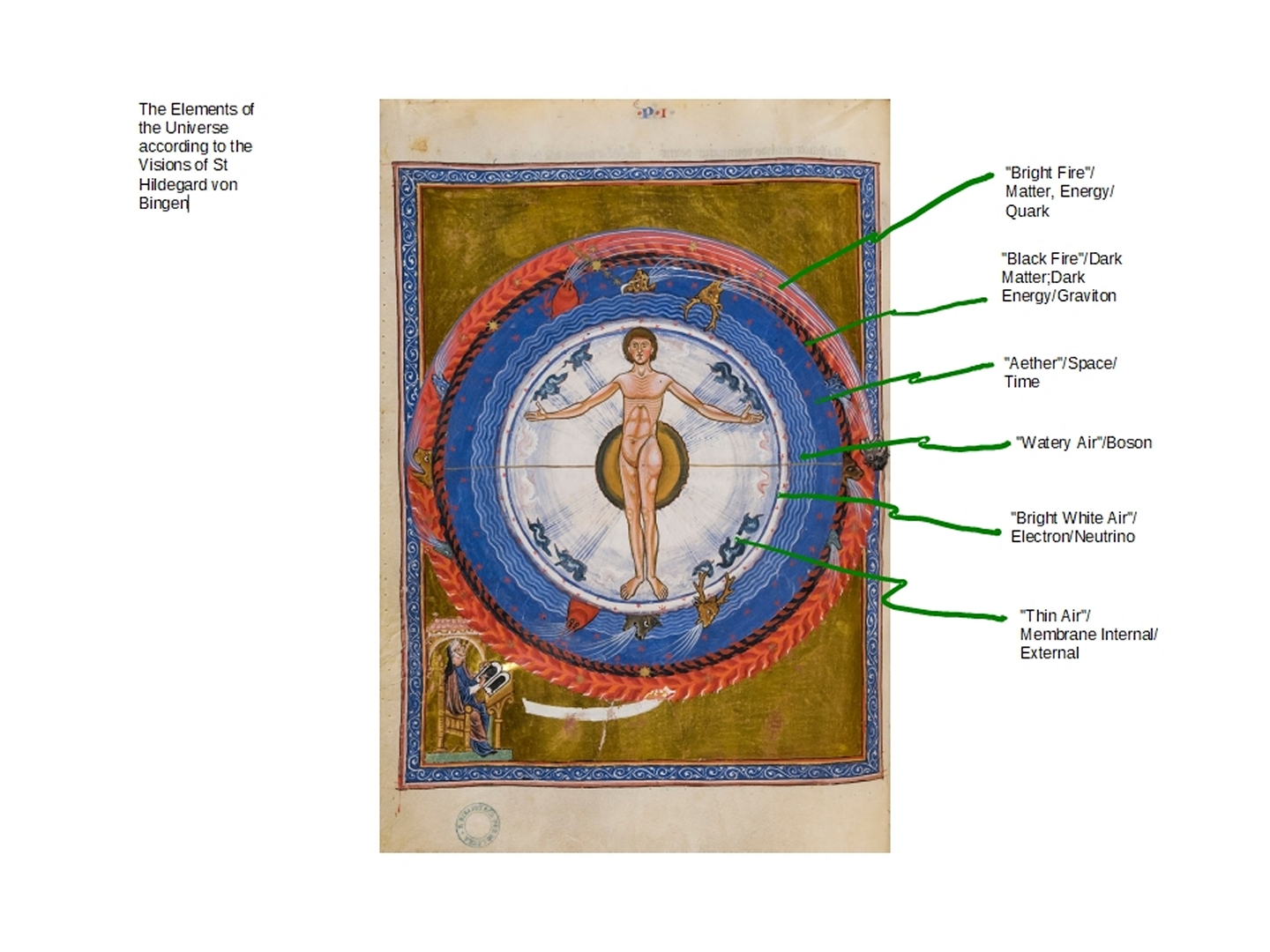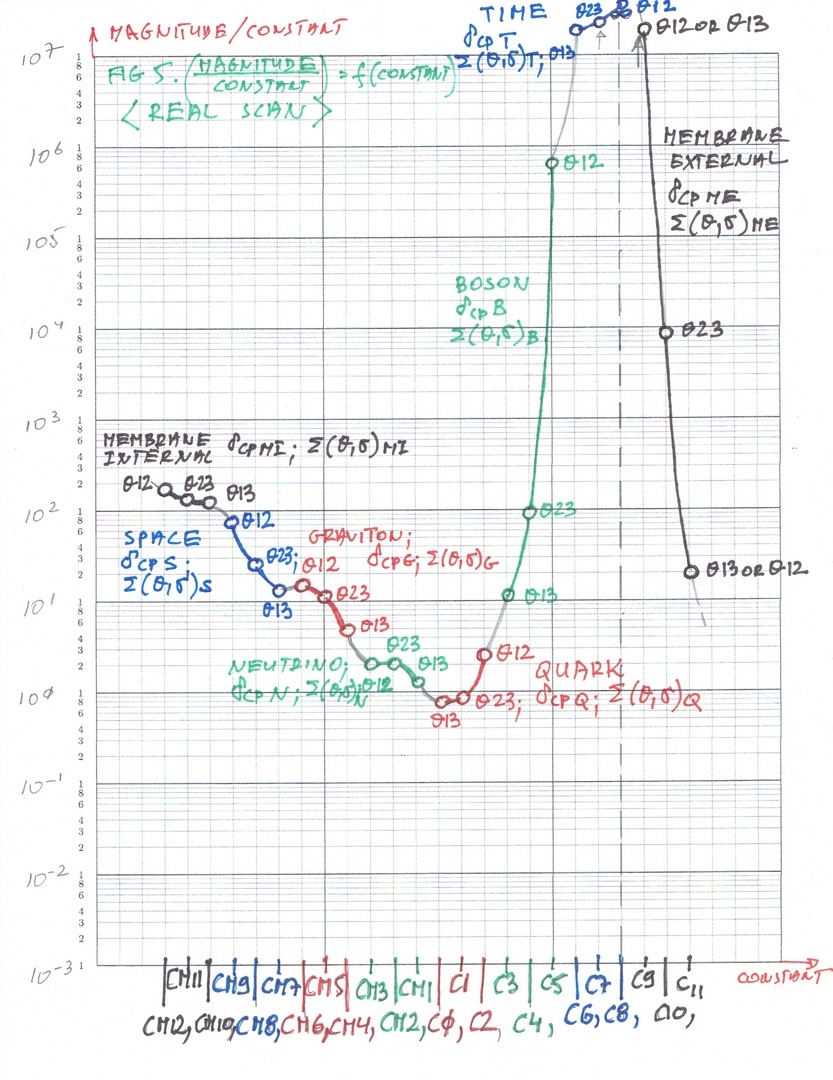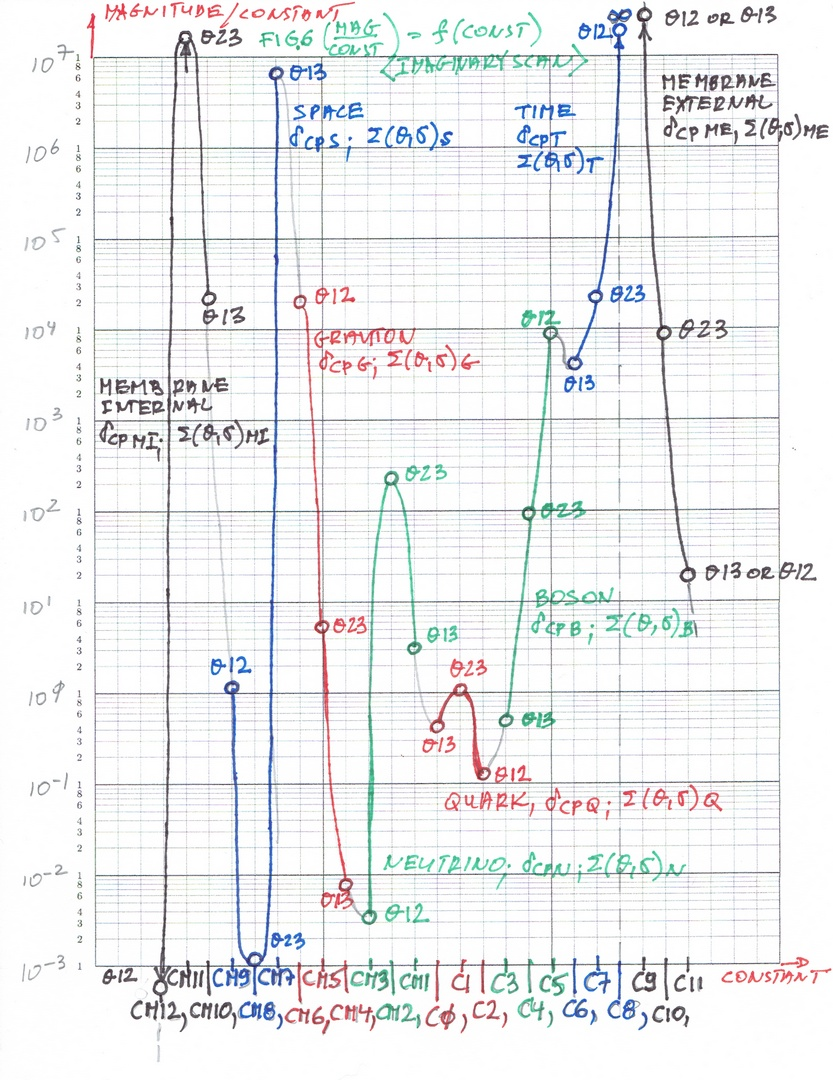24 February 2020 AD; St Matthias (1st Century.)

In this article, I will be presenting three graphs of combined Quantum and Cosmos, according to the vision of St Hildegard von Bingen and according to my calculations. It looks, that the Quantum structure and Cosmos structure are at the same place and can be described by those graphs (and of course by the equations).

On the X-axis of the graphs instead of using real numbers or integers, I am using the transcendental constants numbered from CM12 (smallest) to the biggest - C11. For example, C8 will be π, and C7 will be Euler's number e, etc. You can read more about it in article  4. Transcendental Function and Constants  On the Y-axis instead of using just the results, the results are divided by the respective constant, i.e. if the X value was at the constant C8, then the result on Y will be divided by the constant C8;  if the calculated value was on the C0 (X-axis), then the corresponding result on Y-axis will also be divided by the Transcendental Constant C0, etc. So, one can say instead of using real numbers on the graphs I use transcendentals. This is because I think the universe (by God design) uses a bit different mathematics, a bit more complicated. As you can see from the main equations that mathematics consists of complex exponentials and complex polynomials together in one equation.

The formulas used to get all those values on the graphs are from the article < 16. Integer formula for calculating constants  > You can read also in articles 18 and 19 complete formula for all the values, not just integers (so it is analog as well as discrete) <  18. General formula for calculation of the constants part one    and  <  19. General formula for calculation of the constants part two

It does not really matter because the results are the same, Integer Formula is just a lot simpler, so I had used it, instead of the Final Formula.

The first graph Fig 4. shows the relation of the Sum of the Imaginary and Real scans (more about it later when I present the Fortran programs used to calculate these things). this is the equation on which all the known coupling constants are located as well as known mixing angles for Quark, Neutrino, and one for Boson, plus these results give exact values of the CP Violationg Phase Angles of Quark and Neutrino. In addition, I have calculated all the other mixing (oscillation) angles as well as the CP Violating Phase Angles of all the other elements. And there are plenty of them - 8 sets to be exact. Each set consists of three mixing angles and three other angles (sigma). The sum of all these six angles gives another very useful value which is tied up to the antimatter angle i.e. CP Violating Phase Angle for each element.

The Unity of the Quantum and of the Cosmos is, that all these 8 sets of elements are in the same place for both Quantum and Cosmos, i.e. the forces, such as gravity (dark matter), and force coming from the quarks (probably strong nuclear force) are tied up and the same for Quantum and Cosmos. In other words, the structure of the elements (layers) described by St Hildegard von Bingen as the building blocks of the Universe are in the same place as the building blocks of the Quantum.

So, here is the first graph - Sum of the Imaginary and Real Scans of the main function calculating the values  (magnitudes of a vector) as well as the angles of these vectors. In other words, on X-axis we have Transcendental Constants and on the Y-axis we have the magnitudes/respective constants. For clarity, the mixing angles locations are also included on the graph.Now, the layers of the Universe according to the Vision of St Hildegard von Bingen:Fig 5. This is similar to the graph from Fig 4., except it is probably most clear because it consists only of the Real scan (Real values).

Again we have 8 sets of the elements (forming pairs - more about it in next articles); each set consists of three mixing angles and three sigmas; so we have in each set three pairs, but I think only one pair is stable (such as up and down quark, etc. for other elements).In the next articles, the next and the last graph will be the first attempt of showing that there exists another Universe attached to ours, also consisting of 24 elements but with completely different numerical values than the elements of our Cosmos. It will also show that the one value of the Time Element is undefined, and therefore all dreams about time machine etc. are not possible*. There might be some speculations about the Parallel  Universe and so on. As you probably already figured out a long time ago, I am the Conservative Catholic and for me this another section of the Universe is just Heaven - a place of the angels, saints and of course partly of our Lord God (Heaven can not contain Him).

*Matthew 24:36: "But concerning that day and hour no one knows, not even the angels of heaven, nor the Son, but the Father only." (I will do one day what I call "Mathematical Theology". For now, the undefined value on the constant C8 i.e. π represents God the Eternal Father, "e" - the Euler's Constant represents the Son, Jesus Christ and the ratio of "π/e" is the symbol of the Holy Spirit. "Incidentally " this ratio, this "fraction" is used to compute the Imaginary and Real Scans, giving all those values of thetas (mixing angles), coupling constants and CP Violating Phase angles (matter/antimatter) as well as sigmas (the sums of angles before and after the transformation) precisely.

Fig 6. Imaginary scan only. The Sum of the graph from Fig 6. and the graph from Fig 5. (Real scan) give the first graph - Fig 4. (Imaginary and Real scan)This much in this section. There will be two more articles with graphs - vectors forming spirals (three graphs) and the first attempt of the peak on the other side - the other Universe (you know well my deep Catholic Faith - it might be as well place we call home - Heaven).